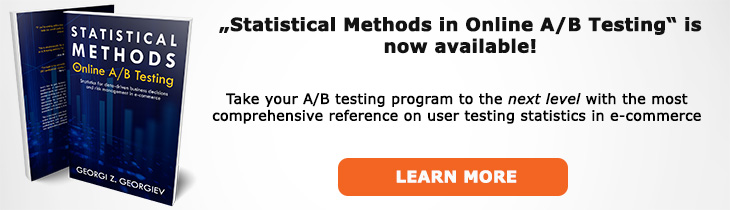# What does "Consistent Estimator" mean?

Definition of Consistent Estimator in the context of A/B testing (online controlled experiments).

## What is a Consistent Estimator?

A consistent estimator in statistics is such an estimate which hones in on the true value of the parameter being estimated more and more accurately as the sample size increases. So for any n0, n1, ... , nx, if nx2 > nx1 then the estimator's error decreases: εx2 < &epsilonx1. For there to be a consistent estimator the parameter variance should be a decreasing function as the sample size increases.

Asymptotic (infinite-sample) consistency is a guarantee that the larger the sample size we can achieve the more accurate our estimation becomes. A notable consistent estimator in A/B testing is the sample mean (with proportion being the mean in the case of a rate).

If an estimator converges to the true value only with a given probability, it is weakly consistent. If convergence is almost certain then the estimator is said to be strongly consistent (as the sample size reaches infinity, the probability of the estimator being equal to the true value becomes 1). Both weak and strong consistency are extensions of the Law of Large Numbers (LLN).

Like this glossary entry? For an in-depth and comprehensive reading on A/B testing stats, check out the book "Statistical Methods in Online A/B Testing" by the author of this glossary, Georgi Georgiev.## Glossary Index by Letter

Select a letter to see all A/B testing terms starting with that letter or visit the Glossary homepage to see all.